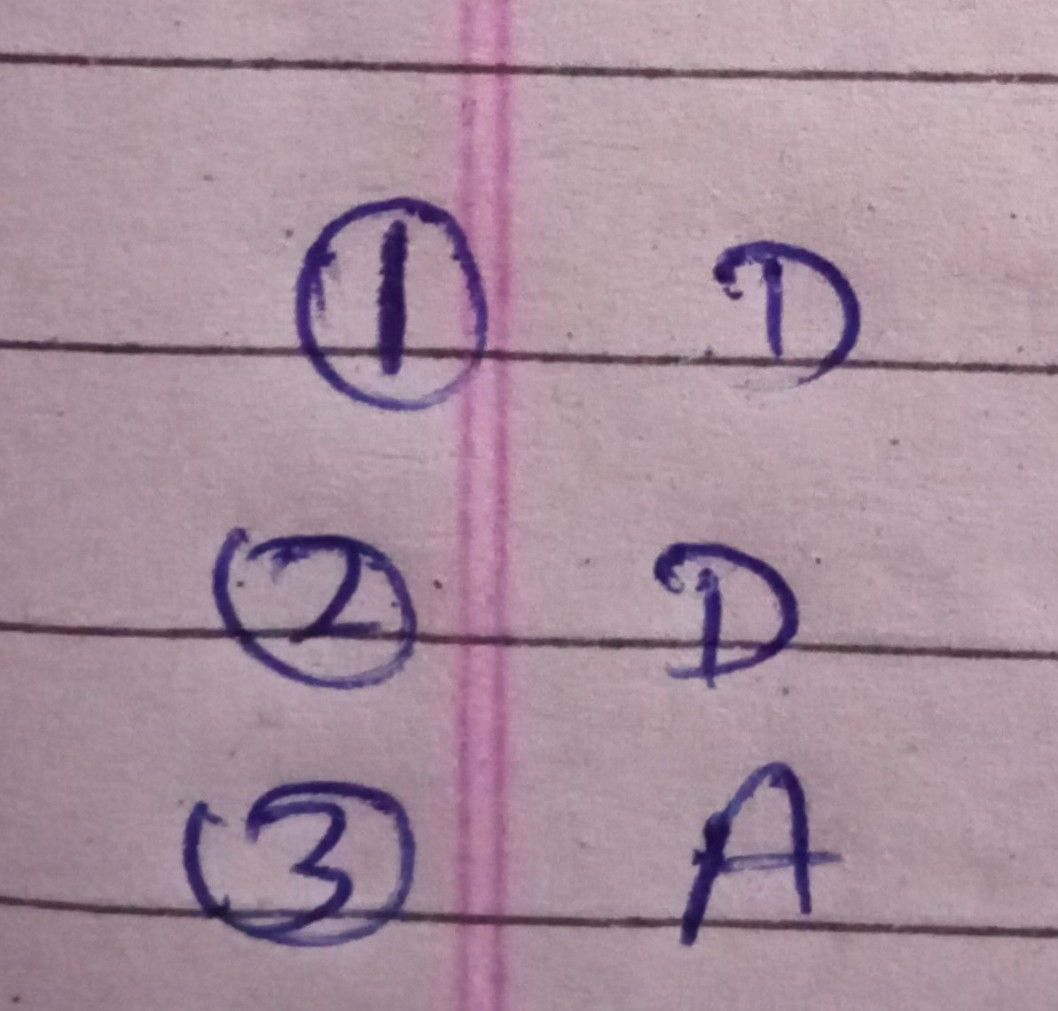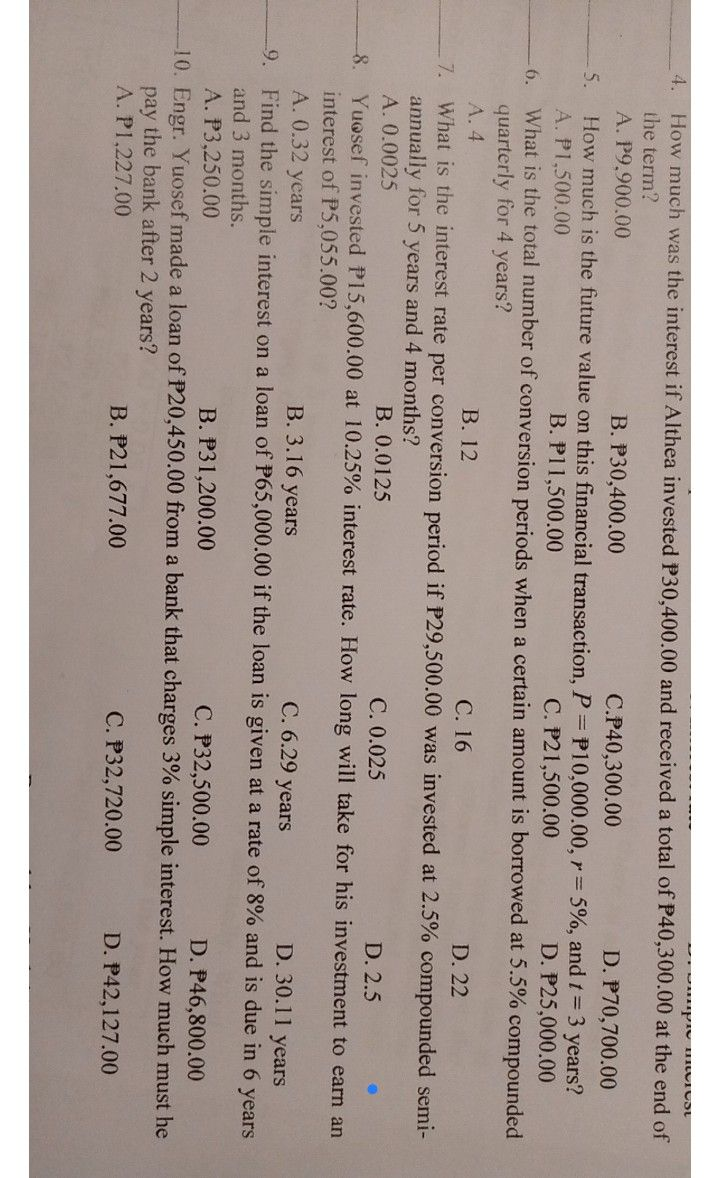Symbol
ProblemDirections: Read each statement carefully. Choose the letter of the correct provided. 1. This refers to the interest charged on the principal alone for the entire duration or period of the loan or investment. A. Compound interest B. Future value C. Interest rate D. Simple interest 2. This refers to the number of years for which the money is borrowed or invested. A. Conversion period B. Interest rate C. Principal D. Time 3. An interest resulting from the periodic addition of simple interest to the principal amount. A. Compound amount B. Compound interest C. Interest rate D. Simple interest 4. How much was the interest if Althea invested P30,400.00 and received a total of P40,300.00 at the end of the term? $r70.700.0$ A. P9,900.00 B. P30,400.00 5. How much is the future value on this financial transaction, $D.m$ $c$ $40.300.00$ $P=p10.000.00,r=590,andt=$ $D.r25$ $50000$ 3 years? A. P1,500.00 B. PI1,500.00 $C.r21.500.00$ $P25.000.00$ number of conversion periods when a certain amount is borrowed at 5.5% compounded p. What is $1etota1num$ $for4ycaos2$ quarterly B. 12 D. 22 A. 4 What is the interest rate per conversion period if $C.16$ $29.500.00was$ invested at 2.5% $comp0undc$ semi- annually for 5 years and 4 months? A. 0.0025 B. 0.0125 D. 2.5 Yuosef invested P15,600.00 at 10.25% interest rate. How lCon. g 0.0w2i5 ll take for his investment to earn an interest of P5,055.00? A. 0.32 years B. 3.16 years $C.6.29ycars$ D. $30.11ycars$ $dnein6ycar$ Find the simple interest on a loan of $P65.000.00ifthc$ loan is given at a rate of 8% and and 3 months. A. P3,250.00 $B.P31.200.00$ C. $P32.500.00$ D. $P46.800.00$ D. Engr. Yuosef made a loan of $\pi omaban$ $P20.450.00f$ that charges 3% simple interest. How much must he pay the bank $ancr2ycas$ A. P1.227.00 $B.r21.677.00$ $C.P32,720.00$ $D.P42.127.00$
10th-13th grade
Other
Search count: 143
SolutionQanda teacher - Ankit264dear student, the remaining questions are based on the formula, so kindly try to solve them.Student
thank youQanda teacher - Ankit264
these are numerical problems, use formulae to solve them,